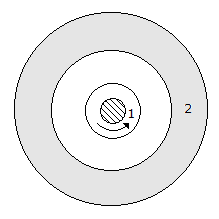# Civil Engineering - UPSC Civil Service Exam Questions

41.

A beam of uniform strength refers which one of the following ?

 A. A beam in which extreme fibre stresses are same at all cross-sections along the length of the beam. B. A beam in which the moment of inertia about the axis of bending is constant at all cross-sections of the beam. C. A beam in which the distribution of bending stress across the depth of cross-section is uniform of all cross-sections of the beam. D. A beam in which the bending stress is uniform at the maximum bending moment cross-section.

Explanation:

No answer description available for this question. Let us discuss.

42.

A sample of ground water at a pH of 7.0 contains 122 mg/l of bicarbonates. What is the alkalinity of this water (in terms of CaC0 ) ?

 A. 120 mg/1 B. 60 mg/1 C. 100 mg/1 D. 200 mg/l

Explanation:

No answer description available for this question. Let us discuss.

43.

The effective depth of a singly reinforced rectangular beam is 30 cm. The section is over-rein-forced and the neutral axis is 12 cm below the top. If the maximum stress attained by concrete is 50 kg/cm2 and the modular ratio is 18, then the stress developed in steel would be

 A. 1800 kg/cm2 B. 1600 kg/cm2 C. 1350 kg/cm2 D. 1300 kg/cm2

Explanation:

No answer description available for this question. Let us discuss.

44.

Soil at a site consists of two layers. The top layer has permeability k units and bottom layer has permeability 5k units. If the thickness of both the layers is equal, then what is the average permeability in the vertical direction ?

 A. 3k units B. (5/3)k units C. (6/5)k units D. (5/6)k units

Explanation:

No answer description available for this question. Let us discuss.

45.

A part of the Newmark's influence chart with four concentric circles is shown in the above figure. If the hatched areas 1 and 2 are loaded separately with the same intensity of loading, then the intensity of pressure yieldedA. By 1 will be more than that yielded by 2 B. By 2 w ill be more than that yielded by 1 C. By 1 and 2 will be equal D. at the centre will be in inverse proportion to the radii of the two, circles